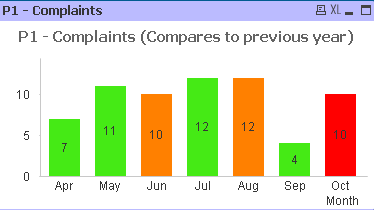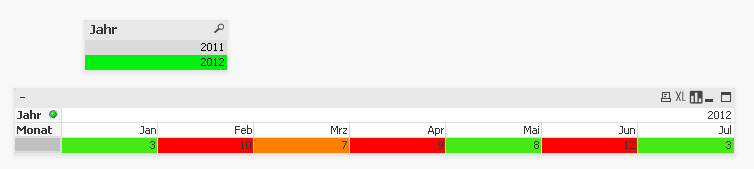# New to QlikView

Discussion board where members can get started with QlikView.

Announcements

#### Breathe easy -- you now have more time to plan your next steps with Qlik! QlikView 11.2 Extended Support is now valid through December 31, 2020. Click here for more information.Not applicable

## issue with Straight table when setting up background color

Hello Friends,

I am facing some issue with Straight table when setting up background color.

i am using the same expression which is used for bar chart for setting the background color. Its working fine with Bar chart( I think its because of the Dimension is

"Month" .

When i use this expression for Straight table, it is displaying the same background color for all the field values. I am not sure, what exactly the problem is?

Expression:

=if (Sum ({\$<Year={\$(#=Only(Year))}>} [P1 - Complaints]) < (Only(If(Ref='P1',RAGGreen)/100) * Sum ({\$<Year={\$(#=Only(Year)-1)}>} [P1 - Complaints])),RGB(69,234,21),if (Sum ({\$<Year={\$(#=Only(Year))}>} [P1 - Complaints]) <= Sum ({\$<Year={\$(#=Only(Year)-1)}>} [P1 - Complaints]), RGB(255,128,0), RGB(255,0,0)) )

Where 'Ref' and 'RAGGreen' are columns from RAG table.

For P1- Complaints, Ref='P1' and RAGGreen=90.

iam comparing the current year compaints with 90% of Previous years complaints in the expression.The columns in the Straight Table are displayed horizontally.

Regards

Siva

New Contributor

## Re: issue with Straight table when setting up background color

with the Pivot-table you cummulate the values according to the dimension Year.

So You have to use Aggr-function:=

aggr(

if (Sum ({\$<Jahr={\$(=(Jahr))}>}Complaints ) < ( 90/100 * Sum ( {\$<Jahr={\$(=Jahr-1)}>} Complaints)),RGB(69,234,21),

if (Sum ({\$<Jahr={\$(=(Jahr))}>} Complaints) <= Sum ({\$<Jahr={\$(=Jahr-1)}>} Complaints), RGB(255,128,0), RGB(255,0,0) ))

,Monat)

Good Luck